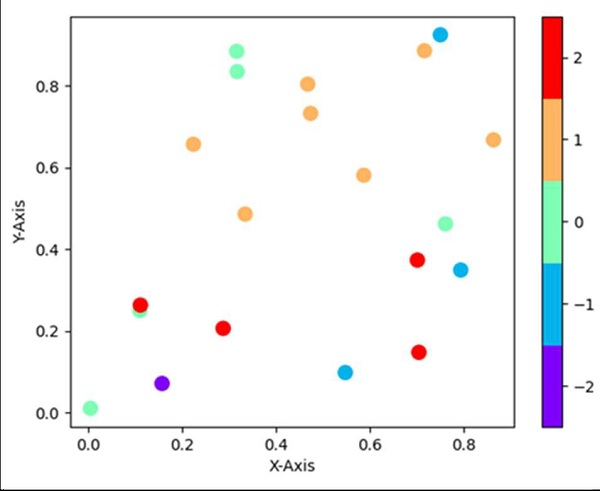# How to make a discrete colorbar for a scatter plot in matplotlib?

Using plt.colorbar(ticks=np.linspace(-2, 2, 5)), we can create a discrete color bar.

## Steps

• Return random floats in the half open interval, i.e., x, using np.random.random method.

• Return random floats in the half open interval, i.e., y, using np.random.random method.

• Return random integers from low (inclusive) to high (exclusive), i.e., z, using np.random.randint(-2, 3, 20) method.

• Set the X-axis label using plt.xlabel().

• Set the Y-axis label using plt.ylabel().

• Use the built-in rainbow colormap.

• Generate a colormap index based on discrete intervals.

• A scatter plot of *y* vs. *x* with varying marker size and/or color, with x, y and z are created (Steps 1, 2, 3).

• Create a colorbar for a ScalarMappable instance, *mappable*.

• Use plt.show() to show the figure.

## Example

from matplotlib import pyplot as plt
import numpy as np
from matplotlib import colors

x = np.random.random(20)
y = np.random.random(20)
z = np.random.randint(-2, 3, 20)

plt.xlabel('X-axis ')
plt.ylabel('Y-axis ')

cmap = plt.cm.rainbow
norm = colors.BoundaryNorm(np.arange(-2.5, 3, 1), cmap.N)

plt.scatter(x, y, c=z, cmap=cmap, norm=norm, s=100, edgecolor='none')
plt.colorbar(ticks=np.linspace(-2, 2, 5))

plt.show()

## Output